Courses

# RD Sharma Solutions -Ex-18.1, Basic Geometrical Tools, Class 6, Maths Class 6 Notes | EduRev

## RD Sharma Solutions for Class 6 Mathematics

Created by: Abhishek Kapoor

## Class 6 : RD Sharma Solutions -Ex-18.1, Basic Geometrical Tools, Class 6, Maths Class 6 Notes | EduRev

The document RD Sharma Solutions -Ex-18.1, Basic Geometrical Tools, Class 6, Maths Class 6 Notes | EduRev is a part of the Class 6 Course RD Sharma Solutions for Class 6 Mathematics.
All you need of Class 6 at this link: Class 6

1.) Construct the following angles using set- squares:

(i) 45o

Place 45o set- square.

Draw two rays AB and AC along the edges from the vertex from the vertex of 45o angle of the set- square.

The angle so formed is a 45o angle.

∠BAC=45o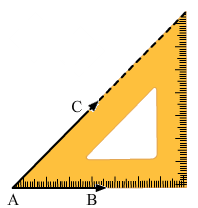(ii) 90o

Place = 90o set –square as shown in the figure.

Draw two rays BC and BA along the edges from the vertex of 90o angle.

The angle so formed is 90o angle.

∠ABC=90o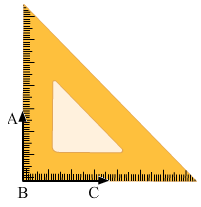(iii) 60o

Place 30o set –square as shown in the figure.

Draw the rays BA and BC along the edges from the vertex of 60o

The angle so formed is 60o

∠ABC=60o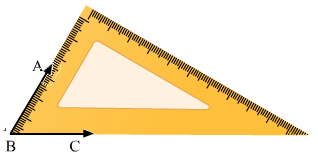(iv) 105o

Place 30o set –square and make an angle 60o by drawing the rays BA and BC as shown in figure.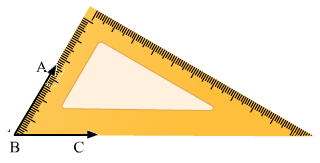Now place the vertex of 45o of the set –square on the ray BA as shown in figure and draw the ray BD.

The angle so formed is 105o

Therefore, ∠DBC=105o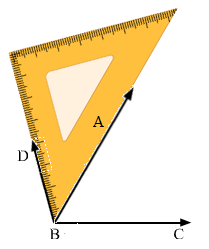(v ) 75o

Place 45o set –square and make an angle of 45o by drawing the rays BD and BC as shown in the figure.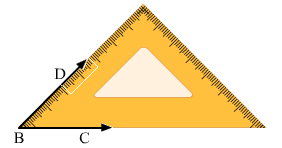Now place the vertex of 30o of the set- square on the ray BD as shown in the figure and draw the ray BA.

The angle so formed is 75o.

Therefore, ∠ABC=75o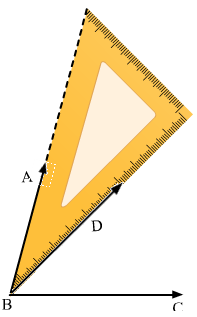(Line BD is hidden)

(vi) 150o

Place the vertex of 45o of the set – square and make angle of 90o by drawing the rays BD and BC as shown in the figure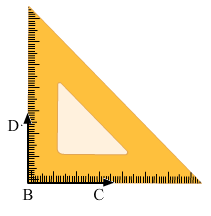Now, place the vertex of 30o of the set –square on the ray BS as shown in the figure and draw the ray BA

The angle so formed is 150o.

Therefore, ∠ABC=150o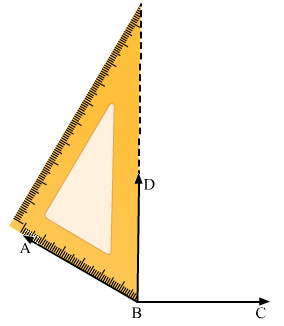2.) Given a line BC and a point A on it, construct a ray AD using set – squares so that ∠DAC is

(i) 30o

(ii) 150o

(i) Draw a line BC and take a point A on it. Place 30o set –square on the line BC such that its vertex of 30o angle lies on point A and one edge coincides with the ray AB as shown in figure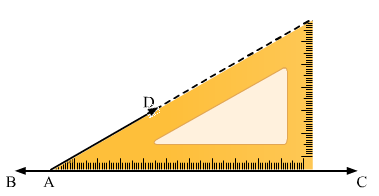Thus ∠DAC is the required angle of 30o

(ii) Draw a line BC and take a point A on it. Place 30o set –square on the line BC such that its vertex of 30o angle lies on point A and one edge coincides with the ray AB as shown in the figure.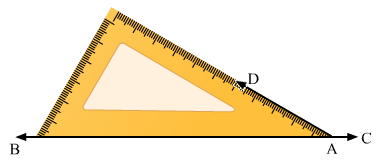Therefore, ∠DAB=30o

We know that angle on one side of the straight line will always add to 180o

Therefore, ∠DAB+∠DAC=180o

Therefore, ∠DAC=150o

103 docs

,

,

,

,

,

,

,

,

,

,

,

,

,

,

,

,

,

,

,

,

,

,

,

,

,

,

,

,

,

,

;